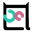# 描边的原理

• 判断面片朝向，找到正反面交汇处的边；
• 将面片沿法向量方向放大，渲染作为描边之后，再次渲染模型；
• 将深度缓冲区或法向量绘制到一张临时纹理，用图像处理的方法，找到突变的地方作为边缘；
• 将法向量接近平行屏幕所在平面的点作为边缘。

# 面片朝向``````遍历模型中的每个三角形：
计算三角形是正面还是反面

如果某条边既包含在正面的三角形中，又包含在反面的三角形中：
将这条边作为描边绘制
``````

## 前向面与后向面

``````N = (V2 - V1) ✕ (V3 - V2)
``````

## 优劣分析# 沿法向量放大## 优劣分析# 图像处理``````void main()
{
float zbuffer = gl_FragCoord.z * gl_FragCoord.w * 100.0;
gl_FragColor = vec4(zbuffer, zbuffer, zbuffer, 1.0);
}
``````

Canny 边缘检测算法，能够得到一个非常理想的边缘——# 平行屏幕方向的法向量

``````varying vec3 vNormal;

void main() {
float silhouette = length(vNormal * vec3(0.0, 0.0, 1.0));
if (silhouette < 0.5) {
silhouette = 0.0;
}
else {
silhouette = 1.0;
}

gl_FragColor = vec4(silhouette, silhouette, silhouette, 1.0);
}
``````# 结合卡通渲染

``````uniform vec3 color;
uniform vec3 light;

varying vec3 vColor;
varying vec3 vNormal;
varying vec3 vLight;

void main()
{
// pass to fs
vColor = color;
vNormal = normalize(normalMatrix * normal);

vec4 viewLight = viewMatrix * vec4(light, 1.0);
vLight = viewLight.xyz;

gl_Position = projectionMatrix * modelViewMatrix * vec4(position, 1.0);
}
``````

``````varying vec3 vColor;
varying vec3 vNormal;
varying vec3 vLight;

void main() {
float silhouette = length(vNormal * vec3(0.0, 0.0, 1.0));
if (silhouette < 0.5) {
silhouette = 0.0;
}
else {
silhouette = 1.0;
}

float diffuse = dot(normalize(vLight), vNormal);
if (diffuse > 0.8) {
diffuse = 1.0;
}
else if (diffuse > 0.5) {
diffuse = 0.6;
}
else if (diffuse > 0.2) {
diffuse = 0.4;
}
else {
diffuse = 0.2;
}
diffuse = diffuse * silhouette;

gl_FragColor = vec4(vColor * diffuse, 1.0);
}
``````